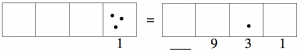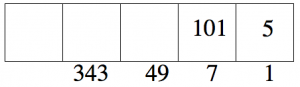$$\newcommand{\id}{\mathrm{id}}$$ $$\newcommand{\Span}{\mathrm{span}}$$ $$\newcommand{\kernel}{\mathrm{null}\,}$$ $$\newcommand{\range}{\mathrm{range}\,}$$ $$\newcommand{\RealPart}{\mathrm{Re}}$$ $$\newcommand{\ImaginaryPart}{\mathrm{Im}}$$ $$\newcommand{\Argument}{\mathrm{Arg}}$$ $$\newcommand{\norm}{\| #1 \|}$$ $$\newcommand{\inner}{\langle #1, #2 \rangle}$$ $$\newcommand{\Span}{\mathrm{span}}$$

2.4: Other Bases

•• Contributed by Michelle Manes
• Professor (Mathematics) at University of Hawaii

$$\newcommand{\vecs}{\overset { \rightharpoonup} {\mathbf{#1}} }$$

$$\newcommand{\vecd}{\overset{-\!-\!\rightharpoonup}{\vphantom{a}\smash {#1}}}$$

In the 1←3 system, three dots in one box is worth one dot in the box one spot to the left. This gives a new picture:Each dot in the second box from the left is worth three ones. Each dot in the third box is worth three 3’s, which is nine, and so on.

Example

We said that the 1←3 code for fifteen is 120. We see that this is correct because:$$1 \cdot 9 + 2 \cdot 3 + 0 \cdot 1 = 15 \ldotp$$

Problem 8

Answer these questions about the 1←3 system.

1. What label should go on the box to the left of the 9 box?
2. What would be the value of a box two spots to the left of the 9 box?
3. What number has 1←3 code 21002?
4. What is the 1←3 code for two hundred dots?

In the 1←4 system, four dots in one box are worth one dot in the box one place to the left.Problem 9

Answer these questions about the 1←4 system.

1. What is the value of each box in the picture above?
2. What is the 1←4 code for twenty-nine dots?
3. What number has 1←4 code 132?

Problem 10

In the 1←10 system, ten dots in one box are worth one dot in the box one place to the left.

1. Draw a picture of the 1←10 and label the first four boxes with their values.
2. What is the 1←10 code for eight thousand four hundred and twenty-two?
3. What number has 1←10 code 95,753?
4. When we write the number 7,842, what does the “7” represent?
The “4” is four groups of what value?
The “8” is eight groups of what value?
The “2” is two groups of what value?
5. Why do you think we use the 1 ← 10 system for writing numbers?

Definition

Recall that numbers written in the 1←2 system are called binary or base two numbers.

Numbers written in the 1←3 system are called base three numbers.

Numbers written in the 1←4 system are called base four numbers.

Numbers written in the 1←10 system are called base ten numbers.

In general, numbers written in the 1←b system are called base b numbers.

In a base b number system, each place represents a power of b, which means $$b^{n}$$ for some whole number n. Remember this means b multiplied by itself n times:

• The right-most place is the units or ones place. (Why is this a power of b?)
• The second spot is the “b” place. (In base ten, it’s the tens place.)
• The third spot is the “$$b^{2}$$” place. (In base ten, that’s the hundreds place. Note that $$10^{2} = 100$$.)
• The fourth spot is the “$$b^{3}$$” place. (In base ten, that’s the thousands place, since $$10^{3} = 1000$$.)
• And so on.

notation

Whenever we’re dealing with numbers written in different bases, we use a subscript to indicate the base so that there can be no confusion. So:

• $$102_{three}$$ is a base three number (read it as “one-zero-two base three”). This is the base three code for the number eleven.
• $$222_{four}$$ is a base four number (read it as “two-two-two base four”). This is the base four code for the number forty-two.
• $$5321_{ten}$$ is a base ten number. (It’s ok to say “fifty-four thousand three hundred and twenty-one.” Why?)

If the base is not written, we assume it’s base ten.

Remember: when you see the subscript, you are seeing the code for some number of dots.

Think / Pair / Share

1. Find the number of dots represented by each of these: $$222_{three} \qquad 310_{four} \qquad 5321_{ten} \ldotp$$
2. Represent nine dots in each base: $$\text{three, five, eight, nine, and eleven} \ldotp$$
3. Which digits are used in the base two system? The base three system? The base four system? The base five system? The base six system? The base ten system?
4. What does the base tell you about the number system? (Think of as many answers as you can!)

Base b to Base Ten

We’re now going to describe some general methods for converting from base b to base ten, where b can represent any whole number bigger than one.

If the base is b, that means we’re in a 1←b system. A dot in the right- most box is worth 1. A dot in the second box is worth b. A dot in the third box is worth , and so on.So, for example, the number $$10123_{b}$$ represents

$$1 \cdot b^{4} + 0 \cdot b^{3} + 1 \cdot b^{2} + 2 \cdot b + 3 \cdot 1,$$

because we imagine three dots in the right-most box (each worth one), two dots in the second box (each representing b dots), one dot in the third box (representing $$b^{2}$$ dots), and so on. That means we can just do a short calculation to find the total number of dots, without going through all the trouble of drawing the picture and “unexploding” the dots.

Example $$\PageIndex{1}$$: Consider the number $$123_{five}$$.

This represents the number $$1 \cdot 5^{2} + 2 \cdot 5 + 3 = 25 + 10 + 3 = 38 \ldotp$$

Example $$\PageIndex{2}$$: Consider the number $$123_{seven}$$.

$$1 \cdot 7^{2} + 2 \cdot 7 + 3 = 49 + 14 + 3 = 66$$

Base Ten to Base b

We’re now going to describe some general methods for converting from base ten to base b, where b can represent any whole number bigger than one.

There are two general methods for doing these conversions. For each method, we’ll work out an example, and then describe the general method. The first method we describe fills in the boxes from left to right.

Example: Method 1 (left-to-right)

To convert $$321_{ten}$$ to a base five number (without actually going through the tedious process of exploding dots in groups of five).

Find the largest power of five that is smaller than 321. We’ll just list powers of five:So we know that the left-most box we’ll use is the 125 box, because 625 is too big.

How many dots will be in the 125 box? That’s the same as asking how many 125’s are in 321. Since

$$2 \cdot 125 = 250 \qquad and \qquad 3 \cdot 125 = 375,$$

we should put two dots in the 125 box. Three dots would be too much.How many dots are left unaccounted for? $$321 - 250 = 71$$ dots are left.

Now repeat the process: The largest power of five that’s less than 71 is $$5^{2} = 25$$. If we put two in the 25 box, that takes care of 50 dots. (Three dots would be 75, which is too much.)So far we have two dots in the $$5^{3}$$ box and two dots in the $$5^{2}$$ box, so that’s a total of

$$2 \cdot 125 + 2 \cdot 25 = 300\; \text{dots} \ldotp$$

We have $$321 - 300 = 21$$ dots left to account for.

Repeat the process again: The biggest power of 5 that’s less than 21 is 5. How many dots can go in the 5 box? $$5 \cdot 4 = 20$$, so we can put four dots in the 5 box.We have one dot left to account for. If we put one dot in the 1 box, we’re done.$$2 \cdot 125 + 2 \cdot 25 + 4 \cdot 5 + 1 = 250 + 50 + 20 + 1 = 321 \ldotp$$

So $$321_{ten} = 2241_{five} \ldotp$$

The general algorithm to convert from base ten to base :

1. Start with your base ten number n. Find the largest power of b that’s less than n, say that power is $$b^{k}$$.
2. Figure out how many dots can go in the $$b^{k}$$ box without going over n. Say that number is a. Put the digit a in the $$b^{k}$$ box, and then subtract $$n - a \cdot b^{k}$$ to figure out how many dots are left.
3. If your number is now zero, you’ve accounted for all the dots. Put zeros in any boxes that remain, and you have the number. Otherwise, start over at step (1) with the number of dots you have left.

The method is a little tricky to describe in complete generality. It’s probably better to try a few examples on your own to get the hang of it.

Think / Pair / Share

Use the method above to convert $$99_{ten}$$ to base three, to base four, and to base five.

Here’s another method to convert base ten numbers to another base, and this method fills in the digits from right to left. Again, we’ll start with an example and then describe the general method.

Example: Method 2 (right-to-left)

To convert $$712_{ten}$$ to a base seven number, imagine there are 712 dots in the ones box. We’ll write the number, but imagine it as dots.Find out how many groups of 7 you can make, and how many dots would be left over.

$$712 \div 7 = 101\; \text{R}5 \; \qquad that\; is,\; 712 = 101 \cdot 7 + 5 \ldotp$$

That means we have 101 groups of 7 dots, with 5 dots left over.

“Explode” the groups of 7 one box to the left, and leave the 5 dots behind.Now repeat the process: How many groups of 7 can you make from the 101 dots?

$$101 \div 7 = 14\; \text{R}3, \qquad meaning\; 101 = 14 \cdot 7 + 3 \ldotp$$

“Explode” the groups of 7 one box to the left, and leave the 3 dots behind.Repeat:

$$14 \div 7 = 2\; \text{R}0, \qquad so\; 14 = 2 \cdot 7 + 0 \ldotp$$

“Explode” the groups of 7 one box to the left, and leave 0 dots behind.Since there are fewer than 7 dots in each box, we’re done.

$$712_{ten} = 2035_{seven} \ldotp$$

Of course, we can (and should!) check our calculation by converting the answer back to base ten:

$$2035_{seven} = 2 \cdot 7^{3} + 0 \cdot 7^{2} + 3 \cdot 7 + 5 = 686 + 0 + 21 + 5 = 712_{ten} \ldotp$$

So here’s a second general method for converting base ten numbers to an arbitrary base b:

1. Divide the base ten number by b to get a quotient and a remainder.
2. Put the remainder in the right-most space in the base b number.
3. If the quotient is less than b, it goes in the space one spot to the left. Otherwise, go back to step (1) and repeat it with the quotient, filling in the remainders from right to left in the base number.

Again, the method probably makes more sense if you try it out a few times.

Think / Pair / Share

Use the method described above to convert $$250_{ten}$$ to base three, four, five, and six.